• 2.回归方程显著性检验 　t 检验（回归系数的检验） 　F 检验（回归方程的检验） 　相关系数的显著性检验 　样本决定系数 　三种检验的关系 一、σ2 的估计 　因为假设检验以及构造与回归模型有关的区间估计...

目 录
1. σ2 的估计
2. 回归方程的显著性检验
t 检验（回归系数的检验）
F 检验（回归方程的检验）
相关系数的显著性检验
样本决定系数
三种检验的关系
一、σ2 的估计
因为假设检验以及构造与回归模型有关的区间估计都需要σ2的估计量，所以先对σ2作估计。
通过残差平方和（误差平方和）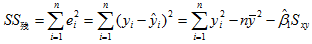（1）

（用到和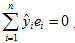，其中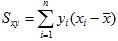）

又∵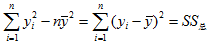（2）
∴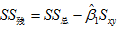（3）
其中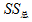为响应变量观测值的校正平方和。残差平方和有n-2 个自由度，因为两个自由度与得到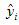的估计值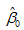与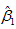相关。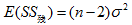（4）
（公式（4）在《线性回归分析导论》附录C.3有证明）
∴  σ2的无偏估计量：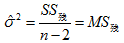（5）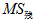为残差均方，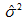的平方根称为回归标准误差，与响应变量y 具有相同的单位。
因为σ2取决于残差平方和， 所以任何对模型误差假设的违背或对模型形式的误设都可能严重破坏σ2的估计值的实用性。因为由回归模型残差算得，称σ2的估计值是模型依赖的。

二、回归方程的显著性检验
目的：检验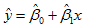是否真正描述了变量 y 与 x 之间的统计规律性。
假设：正态性假设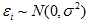（方便检验计算）
t 检验
用t 检验来检验回归系数的显著性。采用的假设如下：
原假设     H0 ： β1 = 0   （x 与 y 不存在线性关系）
对立假设  H1 ： β1 ≠ 0
回归系数的显著性检验就是要检验自变量 x 对因变量 y 的影响程度是否显著。下面我们分析接受和拒绝原假设的意义。
（1）接受 H0 ： β1 = 0  （x 与 y 不存在线性关系）
此时有两种情况，一种是无论 x 取值如何， y 都在一条水平线上下波动，即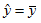，如下图1，另一种情况为， x 与 y 之间存在关系，但不是线性关系，如图2。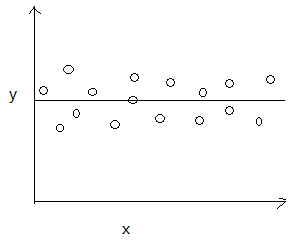图  1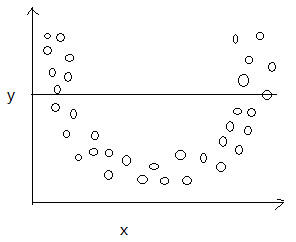图 2
（2）拒绝 H0 ： β1 = 0  （x 对解释 y 的方差是有用的）
拒绝原假设也有两种情况，一种是直线模型就是合适的，如图 3，另一种情况为存在 x 对 y 的线性影响，也可通过 x 的高阶多项式得到更好的结果，如图 4。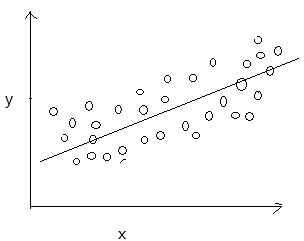图 3图 4
接下来对其检验。
∵（6）
∴ 当H0 ： β1 = 0 成立时，有：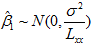（7）在零附近波动，构造 t 统计量：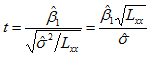（8）
若原假设H0 ： β1 = 0 成立，则，计算|t|，
|t| ≥ tα/2  ， 拒绝 H0
|t| ＜ tα/2  ， 接受 H0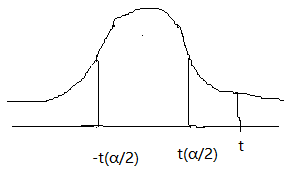2.F 检验（方差分析）
F检验用于检验回归方程的显著性。
方差分析法检验回归显著性，方差分析以分割响应变量 y 的总变异性为基础。
∵∴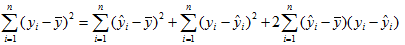∵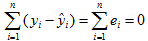，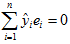∴∴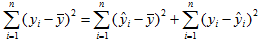（9）
其中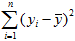称为观测值的校正平方和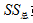或总称为平方和（SST: sum of squares for total,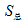， Lyy），其度量了观测值中总的变异性。刻画 y 的波动程度。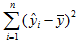称为模型平方和（或回归平方和），记为SSR(R: regression),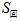,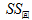。其刻画由 x 的波动引起的 y 波动的部分。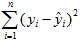是残差平方和（误差平方和），记为SSE(E: error),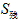,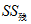。其刻画了未加控制的因素引起 y 波动的部分。
∴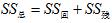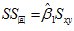（10）
下来分析它们的自由度。因为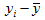约束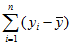使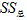丢掉了一个自由度，所以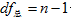个自由度；因为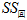完全由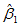一个参数确定，所以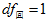个自由度；因为估计β0与β1时对离差施加了两个约束，所以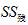有n-2个自由度。
∵ 自由度有可加性
∴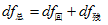（11）
因为总平方和反映因变量 y 的波动程度或称不确定性，在建立了 y 对 x 的线性回归后，总平方和SST就分解成回归平方和SSR与残差平方和SSE这两部分，其中SSR是由回归方程确定的，也就是由自变量 x 的波动引起的，SSE是不能用自变量解释的波动，是由 x 之外的未加控制的因素引起的。这样，总平方和SST 中，能够由自变量解释的部分为SSR，不能由自变量解释的部分为SSE，从而，回归平方和SSR越大，回归的效果就越好，可以据此构造F 检验统计量为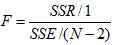(12)
在正态假设下，原假设H0 : β1 = 0 成立时，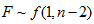，当时，拒绝原假设。
3.相关系数的显著性检验
因为一元线性回归方程讨论的是变量 x 与变量 y 之间的线性关系，所以变量 x 与 y 之间的相关系数来检验回归方程的显著性。用相关系数来反应 x 与 y 的线性关系的密切程度。
x 与 y 的简单相关系数（Pearson 相关系数）：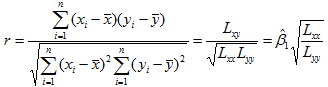（13）
r的绝对值小于等于1：|r| ≤ 1
根据相关系数的检验表，通常当|r| 大于表中α=0.05相应的值时，认为 x 与 y 有显著的线性关系。
缺点：接近于1的程度与数据组数 n 有关（n 较小时，相关系数的波动较大，|r| 接近于1，n 较大时，|r|易偏小，n 较小时，不能仅凭 r 判定 x 与 y 之间有密切的线性关系）
另外补充一点，Pearson相关系数的适用范围：
① 两个变量之间是线性关系，都是连续数据；
② 两个变量的总体是正态分布，或接近正态分布；
③ 两个变量的观测值是成对的，每对观测值之间相互独立。
4.样本决定系数
由公式（10）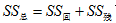可知，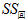越大，线性回归越好，所以定义样本决定系数r2: 回归平方和与总离差平方和之比。（14）
∵（用到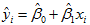和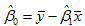）
∴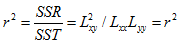（15）
其中最右边的 r2 表示相关系数的平方。
决定系数 r2 是一个回归直线与样本观测值拟合优度（Goodness of Fit, 指回归直线对观测值的拟合程度）的相对指标，反映了因变量的波动中能用自变量解释的比例。
0 ≤  r2  ≤ 1，r2  越接近于 1 ，拟合优度越好。
那么r2 的值比较小的时候反应了什么情况呢？
r2 小的可能原因：
① 线性回归不成立，y 与 x 是曲线关系，应用曲线回归；
② y 与 x 之间符合线性模型，误差项方差σ2大，导致r2 小（n 很大时，线性回归显著）
在对自变量有重复观测时可以通过检验正确区分以上两种不同情况，或者用残差分析的方法。
相关系数和样本决定系数：
相关系数是建立在相关分析的理论基础上，研究两个变量 x 与 y 之间的线性相关关系；样本决定系数是建立在回归分析的理论基础之上，研究非随机变量 x 对 y 的解释程度。样本决定系数除掉了相关系数为0或1（|r|=1 or 0）的情况.
5.三种检验的关系
对于一元线性回归来说，回归系数显著性的 t 检验，回归方程显著性的 F 检验，相关系数显著性的 t 检验，这三种检验是等价的。相关系数显著性的 t 检验与回归系数显著性的 t 检验是完全相等的，式（12）F统计量则是这两个 t 统计量的平方。对于一元线性回归只需要做一种检验即可，而对于多元线性回归，这三种检验考虑的问题不同，是三种不同的检验，并不等价。

转载于:https://www.cnblogs.com/datamining-bio/p/9502033.html
展开全文• 题目 程序及结果 1)程序 x<-c(16,23,32,31,37,40,46,48,55,60) y<-c(13,15,16,17,17,18,19,21,22,24) A<-data.frame(x,y) plot(x,y) lm.reg<-lm(y~x) abline(lm.reg) print(lm.reg) ... 8.97
题目程序及结果
1)程序
x<-c(16,23,32,31,37,40,46,48,55,60)
y<-c(13,15,16,17,17,18,19,21,22,24)
A<-data.frame(x,y)
plot(x,y)
lm.reg<-lm(y~x)
abline(lm.reg)
print(lm.reg)

1)结果
Call:
lm(formula = y ~ x)

Coefficients:
(Intercept)            x
8.9711       0.2379

2)程序
summary(lm.reg)

2)结果
Call:
lm(formula = y ~ x)

Residuals:
Min       1Q   Median       3Q      Max
-0.91258 -0.55828  0.08493  0.59832  0.75740

Coefficients:
Estimate Std. Error t value Pr(>|t|)
(Intercept)  8.97109    0.67400   13.31 9.70e-07 ***
x            0.23786    0.01645   14.46 5.12e-07 ***
---
Signif. codes:  0 ‘***’ 0.001 ‘**’ 0.01 ‘*’ 0.05 ‘.’ 0.1 ‘ ’ 1

Residual standard error: 0.6842 on 8 degrees of freedom
Multiple R-squared:  0.9631,	Adjusted R-squared:  0.9585
F-statistic:   209 on 1 and 8 DF,  p-value: 5.124e-07

3)程序
point<-data.frame(x=45)
lm.pred<-predict(lm.reg,point,interval="prediction",level=0.95)
lm.pred

3)结果
       fit      lwr      upr
1 19.67472 18.00331 21.34613

结果分析展开全文• 多元线性回归显著性检验Matlab程序完美版 一说明 1本程序是硕士教材数理统计杨虎刘琼钟波 编著 例4.4.1P133Matlab编程解答程序教材上例题只做了回归方程显著性分析和一次回归系数显著性分析剔除x1后没有再检验x2和...
• 这学期正在用《应用回归分析（第四版）》，但很多地方语焉不详，例如一元线性回归中的F检验和t检验检验统计量的证明。 以下的证明主要参考了科学出版社出版的《应用回归分析》 检验统计量的推导 t统计量需要...
这学期正在用《应用回归分析（第四版）》，但很多地方语焉不详，例如一元线性回归中的F检验和t检验的检验统计量的证明。 以下的证明主要参考了科学出版社出版的《应用回归分析》
检验统计量的推导
t统计量需要满足的假设：
分子：标准正态分布的随机变量

X

X

分母：卡方分布随机变量

Y

Y

X

X

和

Y

Y

相互独立
F统计量需要满足的假设：
分子和分母均为卡方分布的随机变量，记为

X

X

和

Y

Y

X

X

和

Y

Y

相互独立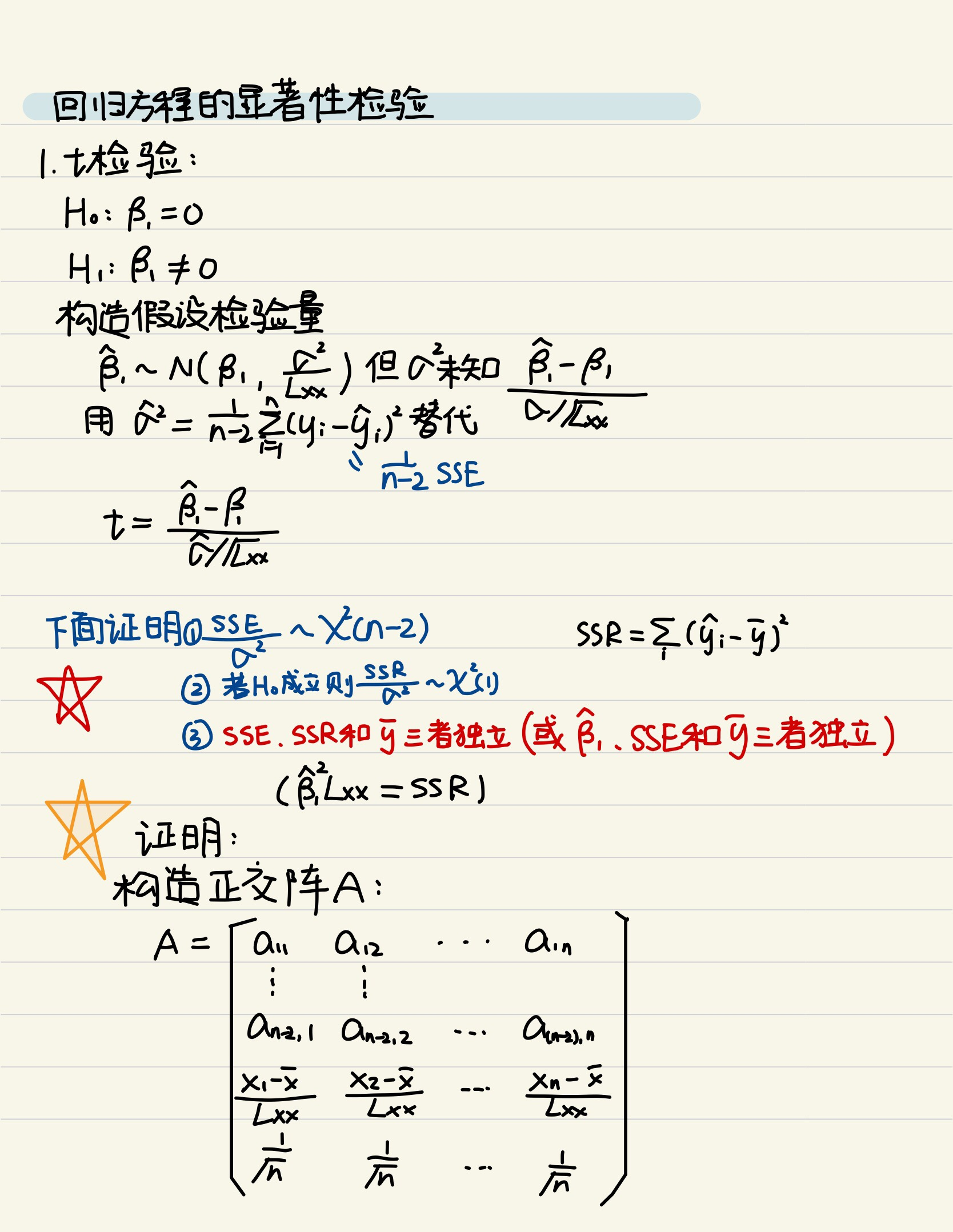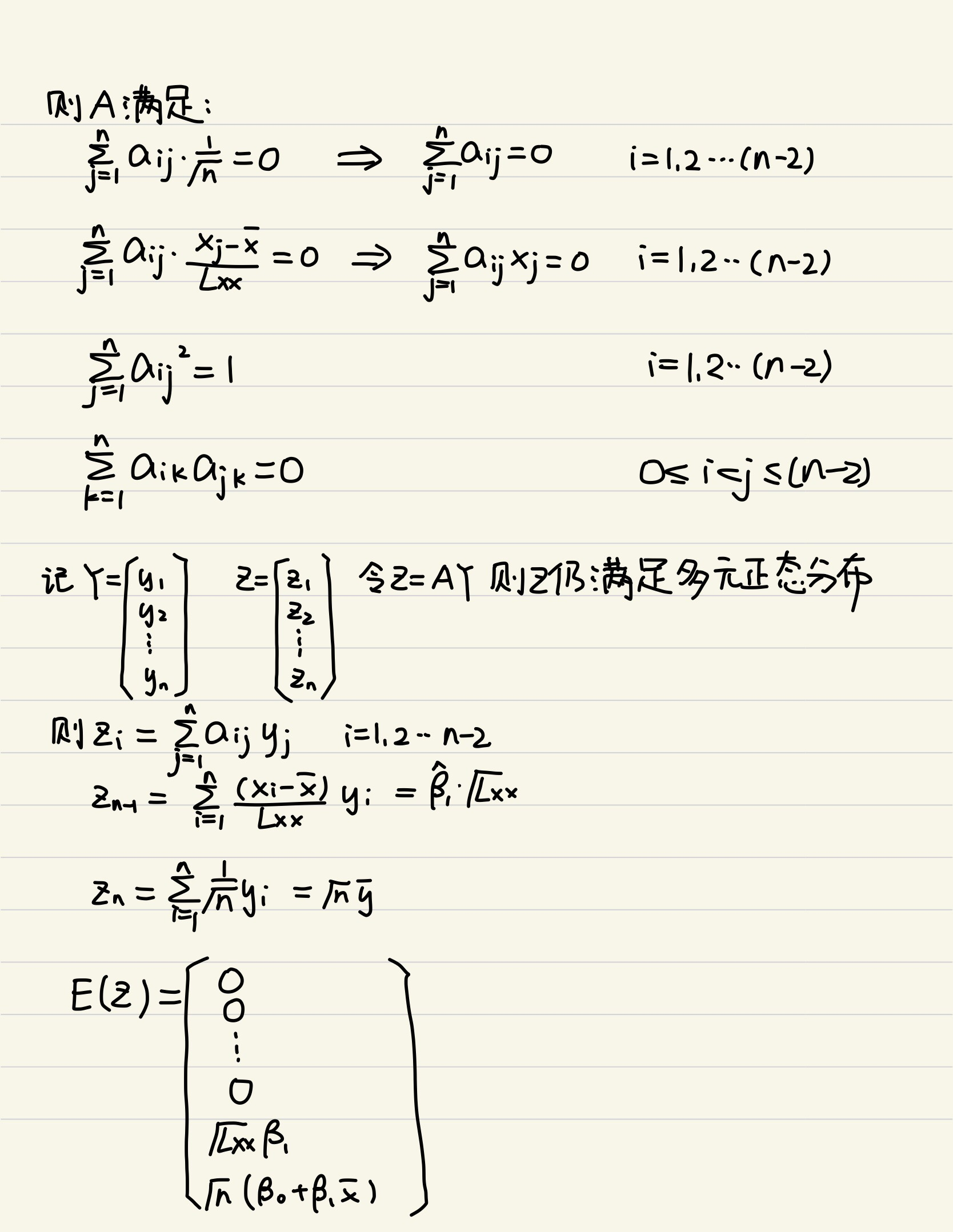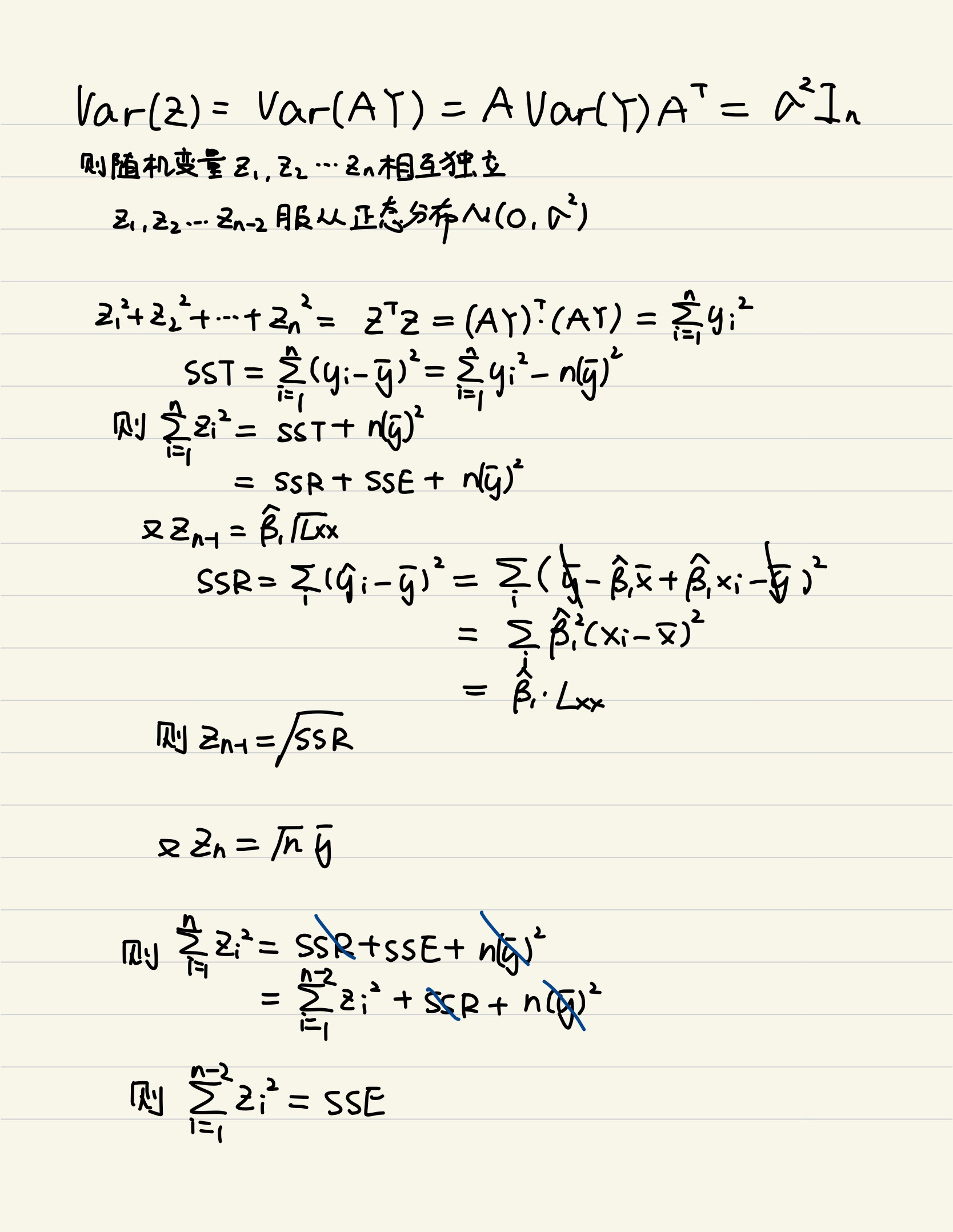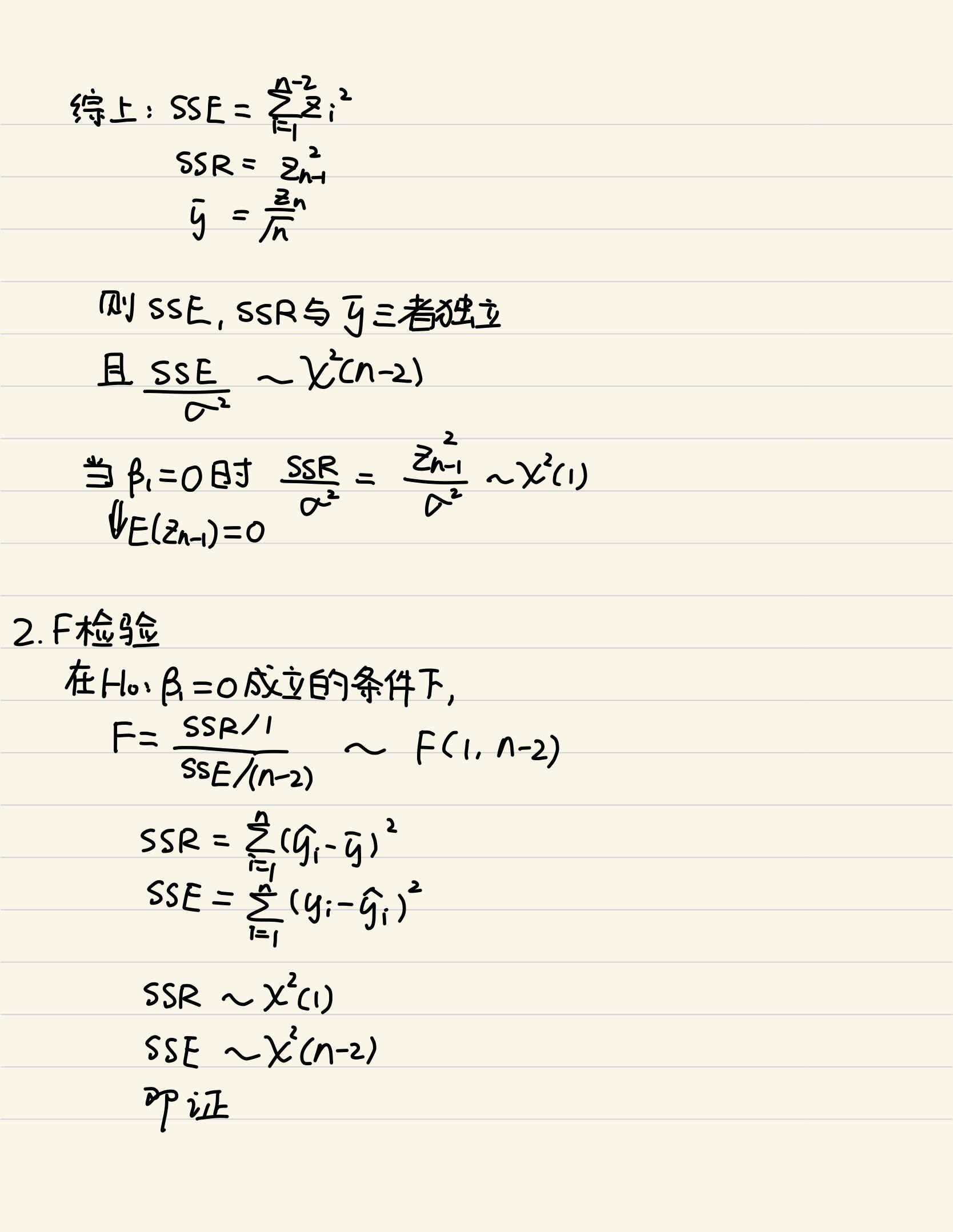展开全文•   假设变量x1,x2,x3,…,xpx_{1},x_{2},x_{3},\dots,x_{p}x1​,x2​,x3​,…,xp​与随机变量yyy之间存在较显著的相关关系，则就有以下的回归模型：y=f(x1,x2,x3,…,xp)+ϵy=f(x_{1},x_{2},x_{3},\dots,

本篇博客的重点不在于回归模型的建立，而在于模型建立后的显著性检验。

1.回归分析
1.1 定义
回归分析就是对具有相关关系的两个或两个以上变量之间数量变化的一般关系进行测定，确定因变量和自变量之间数量变动的数学表达式，以便对因变量进行估计或预测的统计分析方法。回归分析的数学描述如下：   假设变量

x

1

,

x

2

,

x

3

,

…

,

x

k

x_{1},x_{2},x_{3},\dots,x_{k}

与随机变量

y

y

之间存在较显著的相关关系，则就有以下的回归模型：

y

=

f

(

x

1

,

x

2

,

x

3

,

…

,

x

k

)

+

ϵ

y=f(x_{1},x_{2},x_{3},\dots,x_{k})+\epsilon

其中

ϵ

\epsilon

为随机变量。而常用的回归模型是以下的线性形式：

y

=

β

0

+

β

1

x

1

+

β

2

x

2

+

⋯

+

β

k

x

k

+

ϵ

y=\beta_{0}+\beta_{1}x_{1}+\beta_{2}x_{2}+\cdots+\beta_{k}x_{k}+\epsilon

1.2 步骤
(1)根据研究目的和现象之间的内在联系，确定自变量和因变量。   做回归分析时，应该首先从理论出发进行定性分析，根据现象的内在联系确定变量之间的因果关系，从而确定哪个为自变量，哪个为因变量。 (2)确定回归分析模型的类型及数学表达式。   根据现象之间的内在影响机制或通过对具体变量数据描点分析，找出最适合的回归分析模型，再通过计算求出模型的待估参数，得到回归方程。常用的估计方法有普通最小平方法、岭回归、偏最小平方法回归、约束最小平方法回归，主成分回归。 (3)对回归分析模型进行评价和诊断。   得到具体的回归方程以后，要对其进行统计检验，来对回归方程的代表性及拟合程度进行拟合。
2.回归方程的统计检验
对于所拟合的回归模型，需要检验其合理性。检验的内容包括：模型的参数取值含义是否符合经济意义，模型的参数在统计意义上是否显著，模型整体的拟合效果是否理想，模型的假设条件是否满足。
2.1 模型整体拟合效果的显著性检验
记实际观察值为

y

i

y_{i}

，回归方程拟合的理论值(预测值)为

y

c

i

y_{c_{i}}

,观察值的平均值为

y

‾

\overline{y}

,则在最小平方法之下

y

c

‾

\overline{y_{c}}

与

y

‾

\overline{y}

是相等的。根据方差分析原理，则有

∑

(

y

−

y

‾

)

2

=

∑

(

y

c

−

y

‾

)

2

+

∑

(

y

−

y

c

)

2

\sum(y-\overline{y})^{2}=\sum(y_{c}-\overline{y})^{2}+\sum(y-y_{c})^{2}

或

S

S

T

=

S

S

R

+

S

S

E

SST=SSR+SSE

在误差项服从正太分布的情况下，应该有

S

S

R

∼

χ

2

(

k

)

,

S

S

E

∼

χ

2

(

n

−

k

−

1

)

SSR\sim\chi^{2}(k),SSE\sim\chi^{2}(n-k-1)

所以有

F

F

统计量

F

=

S

S

R

/

k

S

S

E

/

(

n

−

k

−

1

)

∼

F

(

1

,

n

−

k

−

1

)

F=\frac{SSR/k}{SSE/(n-k-1)}\sim F(1,n-k-1)

显然，回归方程拟合效果越好，表明方程解释部分所占比重越大，

S

S

R

SSR

和

S

S

E

SSE

相比的值也越大，

F

F

统计量也越大。因此，方程整体显著性检验的假设为：

H

0

:

β

0

=

β

1

=

β

2

=

⋯

=

β

k

=

0

H_{0}:\beta_{0}=\beta_{1}=\beta_{2}=\dots=\beta_{k}=0

H

1

:

β

0

≠

0

或

β

1

≠

0

或

…

或

β

k

≠

0

H_{1}:\beta_{0}\neq0或\beta_{1}\neq0或\dots或\beta_{k}\neq0

再给定显著性水平

α

\alpha

下，若

F

>

F

α

(

1

,

n

−

2

)

F>F_{\alpha}(1,n-2)

则拒绝原假设，认为回归方程整体是显著的。
2.2 模型参数显著性检验
模型参数显著性检验主要是判断每一个自变量对于回归模型是否必要。对于回归方程的单个参数进行

t

t

检验，对于回归方程中的每个参数

β

i

\beta_{i}

，其假设如下：

H

0

i

:

β

i

=

0

,

i

=

0

,

1

,

…

,

k

H_{0i}:\beta_{i}=0,i=0,1,\dots,k

H

1

i

:

β

i

≠

0

,

i

=

0

,

1

,

…

,

k

H_{1i}:\beta_{i}\neq0,i=0,1,\dots,k

其

t

t

检验统计量为：

t

=

β

i

σ

^

y

x

c

i

i

∼

t

(

n

−

k

−

1

)

t=\frac{\beta_{i}}{ \widehat \sigma_{yx}\sqrt{c_{ii}}}\sim t(n-k-1)

其中

c

i

i

c_{ii}

为矩阵

(

X

T

X

)

−

1

(X^{T}X)^{-1}

对角线上第

i

i

个元素，

σ

^

y

x

\widehat \sigma_{yx}

的计算公式如下：

σ

^

y

x

=

∑

(

y

−

y

c

)

2

n

−

k

−

1

\widehat \sigma_{yx}=\sqrt{\frac{\sum(y-y_{c})^{2}}{n-k-1}}

python中的statsmodel包中已经集成了完整的回归方程显著性检验。具体示例如下：
import pandas as pd
from scipy import stats
import statsmodels.api as sm
import numpy as np

X=pd.DataFrame(boston.data,columns=boston.feature_names)
y=pd.DataFrame(boston.target,columns=['target'])

model=sm.OLS(y,X)
results=model.fit()
y_pred=pd.DataFrame(model.predict(results.params,X),
columns=['pred'])
print(results.summary())

"""
自写代码计算过程
SSR=np.dot(y_pred['pred']-y['target'].mean(),y_pred['pred']-y['target'].mean())
SSE=np.dot(y_pred['pred']-y['target'],y_pred['pred']-y['target'])

(1)回归方程显著性检验
f_val=(SSR/13)/(SSE/492)
f_pval=stats.f.sf(f_val,13,492)

(2)单个参数的显著性检验
ttest_result=pd.DataFrame(None,index=X.columns,columns=['tval','pval'])
error=np.dot(y['target']-y_pred['pred'],y['target']-y_pred['pred'])
S = np.array(np.linalg.inv(np.dot(np.mat(X).T,X)))
for i,col in enumerate(X.columns):
tval=results.params[col]/np.sqrt((error/492)*S[i][i])
ttest_result.loc[col,'tval']=tval
pval=stats.t.sf(np.abs(tval),df=492)*2
ttest_result.loc[col,'pval']=pval
"""

其结果如下：从上述试验结果中可以发现，变量INDUS和AGE对回归方程是不显著的，可以从回归方程中剔除这两个变量。
参考资料
https://www.cnblogs.com/tangxianwei/p/8323495.htmlhttps://blog.csdn.net/u011517132/article/details/105286113
展开全文• matlab建立多元线性回归模型并进行显著性检验及预测问题例子;x=[143 145 146 147 149 150 153 154 155 156 157 158 159 160 162 164]'; X=[ones(16,1) x]; 增加一个常数项 Y=[88 85 88 91 92 93 93 95 96 98 97 96 ...
• 1、demo package com.math.demo; import java.math.BigDecimal; import com.math.regression.RegressionEquation; import com.math.regression.RegressionModel;... public static void main(String[] argjava
• ## Python_一元线性回归及回归显著性

万次阅读 多人点赞 2018-09-22 16:07:11
数据源自《应用回归分析》（第四版） ## 火灾损失表 ### 距离消防站km x = [3.4, 1.8, 4.6, 2.3, 3.1, 5.5, 0.7, 3.0, 2.6, 4.3, 2.1, 1.1, 6.1, 4.8, 3.8] ### 火灾损失 千元 y = [26.2, 17.8, 31.3, 23.1, ...python 数据挖掘 机器学习
• % H=1，线性回归方程显著(好)；H=0，回归不显著 % 回归系数的显著性检验 chi2=sqrt(diag(C)*Q/(n-p)); % 服从χ2(n-p)分布 tV=beta_hat./chi2; % 服从T分布，绝对值越大线性关系显著 tInv=tinv(0.5+alpha/2,n-p); ...
• 本文主要讨论Python实现一元回归的线性拟合、最小二乘法估计回归参数和显著性检验（t检验和p值）.一元线性回归模型是描述两个变量之间相关关系的最简单的回归模型. 通常人们对所要研究的问题首先要收集与它有关的n组...
• 转载于：回归方程显著性检验（F检验）是单侧还是双侧检验，为什么？
• 回归方程显著性检验如何进行？具体程序是什么？ 实验数据如下： X Y C 1100 57.66 1.8 1157 57.79 1.7 1272 58.03 1.67 1330 58.22 1.67 1389 57.69 1.7 1590 57.01 1.67 1820 55.42 1.6 2049 59....
• 本文主要讨论Python实现一元回归的线性拟合、最小二乘法估计回归参数和显著性检验（t检验和p值）. 一元线性回归模型是描述两个变量之间相关关系的最简单的回归模型. 通常人们对所要研究的问题首先要收集与它有关的n...Python 最小二乘法 p值
• %%1、bint表示回归系数区间估计 %2、r表示残差 %3、rint代表置信区间 ...% r^2越接近于1，回归方程显著 %alpha表示显著水平 %% x=[143 144 145 147 148 150 153 154 155 156 157 158 159 160 1...matlab
• 回归方程的预报精度 回顾总结 十一、回归方程与回归系数的显著性检验 回归方程的显著性检验，检验的是我们建立线性回归方程的合理性，因为我们不能肯定模型是正确的，也就是说我们需要检验 YYY与 x1,⋯ ,xmx_1,\...多元统计分析
• 检验回归效果显著性 1）设 H 0 : b = 0 , H 1 : b ≠ 0 H_0:b=0,H_1:b\not =0 H 0 ​ : b = 0 , H 1 ​ : b  ​ = 0 2）判断 ∣ b ^ ∣ σ ^ S x x 与 t α 2 ( n − 2 ) \frac{|\hat b|}{\hat \sigma}\sqrt{S_...
• 　在研究某一现象时，主要关心与影响最主要因素关系时，两者有密切关系，但并非一个变量唯一确定另一个变量，可以使用一元线性回归方程 　 　 　回归分析的任务：通过n组样本的观察值，对β进行估计，得到最终的...
• 一元线性回归一元线性回归模型一元线性回归的参数估计最小二乘估计最大似然估计最大似然估计数学推导有偏估计与无偏估计参数估计的性质一元线性回归显著性检验一元线性回归的残差分析一元线性回归模型的应用 一元...
• 一元线性回归模型的概念 这也要从一个女装公司讲起！ 问：请用函数关系描述身高与腿长的联系。 一般接受过培训懂点数模的同学应该非常清楚的是，拿到此题，先画出散点图，观察是什么样子的，然后进行下一步观测！ ...
• 采用一元线性回归分析和最小二乘法，逐像元拟合近n年间遥感影像每个栅格NDVI的斜率Slope，得到多年NDVI的变化趋势，综合分析流域多年植被覆盖变化额方向和速率。 式中，slope为变化趋势；yi为第xi年的值；当slope...
• 目录导引Chap 1 一元线性回归1.1 基本模型与假设1.2 参数β0,β1\beta_0,\beta_1β0​,β1​的估计与性质1.3 回归方程显著性检验以及检验等价1.3.1 β1\beta_1β1​的t检验1.3.2 回归方程的显著性F检验1.3.3 相关...统计学 统计模型
• 通过取若干组试件达到极限破坏状态时的法向力σ和切向力τ,建立σ关于τ的一元线性回归方程,并编写了相应程序解得最佳c、φ值,进一步对回归方程进行检验,最终发现当取显著性水平α≥0.005时,τ与σ之间有线性关系,当...
• 回归分析中，如果有两个或两个以上的自变量，就称为多元回归。...例如，家庭消费支出，除了受家庭可支配收入的影响外，还受诸如家庭所有财富、物价水平、金融机构存款利息等多种因素的影响，表现在线性回归模型中的解matlab 多元线性回归
• 2.线性方程的相关计算 x=[1,2,3,4,5]';%参数矩阵 X=[ones(5,1),x];%产生一个5行一列的矩阵，后接x矩阵 Y=[3.95,5.23,7.6,9.48,11.89]'; [b,bint,r,rint,stats]=regress(Y,X) %b为方程相关系数 ...
• 模型显著性检验 相关系数实现代码 x=c(1,2,3,4,5) y = c(1,1.5,3,4.5,5) n=5 xy=data.frame(x,y) cor=cor.test(x,y) plot(x,y) x.mean=mean(x) y.mean=mean(y) Sxy=sum((x-x.mean)*(y-y.mean)/(n-1)...最小二乘法 R语言lm
• 文章目录基本概念最小二乘法统计学方法统计学建模统计学方法估计a和b估计均方误差线性假设的显著性检验置信区间以及预测区间 基本概念 从独立的两个随机变量X和Y中获取n对观察结果组成的样本(X1,Y1),(X2,Y2),…,(Xn,...数据分析...